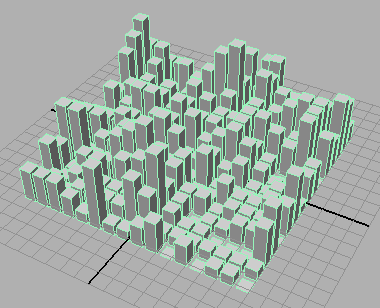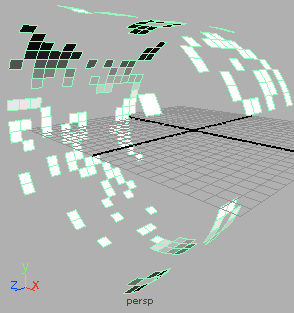### Cellular Automata PolyPlanes

#### Introduction

The tutorial, "Algorithmic Form: Basic CA Python Classes", presented two python classes that implement the logic that underpins the classes developed in this tutorial. The two classes presented in listing 1, CellularPlanes.py, enable a cellular automata to be modelled in Maya as a grid of instanced shapes - figure 1.Figure 1 - 20 by 20 cells [click to play]

#### The MayaCell Class

This is a subclass of `AbstractAutomata.Cell`. It has four class variables that are shared by all instances of the class. For efficiency, each instance of `MayaCell` uses Maya's `instance()` proc to create a "copy" of single "master" object ie.

`    self.tformNode = mc.instance(MayaCell.shape)`

By default, the master shape is a Maya polyPlane and the attribute that responds to the state of a `MayaCell` is the visibility of the shape. MayaCell overrides the `__init__` method of its super class in order to create a Maya instance and to set its pivot point.

```   def __init__(self, chance):
# Call the super class init method
CA.Cell.__init__(self, chance)
self.tformNode = mc.instance(MayaCell.shape)
mc.xform(self.tformNode, piv = MayaCell.pivot)    ```

Setting the pivot is of no importance to a polyPlane but it is important when other Maya shapes are used. For example, before an instance of the `MayaAutomata` class is created the class variables of `MayaCell` can be assigned new values ie.

```mc.delete(MayaCell.shape)
MayaCell.shape = mc.polyCube(w = 0.8, h = 0.8, d = 0.8)
MayaCell.attrName = ".scaleY"
MayaCell.attrIncr = 1
MayaCell.pivot = (0, -0.4, 0)```

`MayaCell` has been written so that a variety of graphics can be produced easily. For example, figure 2 shows the effect of using a polyCubes whose scaleY attribute is "driven" by the number of times a cell was "alive" during 10 generations of the automata.Figure 2

The setState() method enables a variety of potentially interesting visual effects to be easily achieved (figures 3 & 4). If the reader wishes the Maya shape that represents a cell to have more than one attribute effected by a cell's state they should create their own subclass of AbstractAutomata.Cell.

 Figure 3 Figure 4

#### The MayaAutomata Class

The class ensures that instances of `MayaCell`, rather than the default `Cell` class are used for the automata ie.

```    # This overrides the base class so that an instance
# of our custom class can be used by the automata. It
# is called by the Automata.__init__() method.
def getACell(self, chanceOfLife):
cell = MayaCell(chanceOfLife)
MayaAutomata.listOftNodes.append(cell.tformNode)
return cell```

The class is responsible for laying out the Maya shapes that represent the cells of the automata. Although the initGraphics() method lays out the shapes in a grid there is no reason why they cannot be positioned in more interesting configurations. For example, figure 5 used this slightly modified version of the method.

```    def initGraphics(self):
count = 0

angle = 360.0/self.rows
print "\n"
print angle

for i in range(self.cols):
x = startAt
#y = 0
z = startAt + i
for j in range(self.rows):
cell = self.cells[i][j]
mc.xform(cell.tformNode, piv = (0, -radius, 0),
ro = (0, 0, j * angle),
mc.setAttr((MayaCell.shape + ".visibility"), 0)
MayaAutomata.listOftNodes.append(MayaCell.shape)
mc.group(MayaAutomata.listOftNodes)```Figure 5 - 20 by 20 cells [click to play]

The classes have been implemented on the assumption the reader wishes to animate the cells over an arbitary number of generations ie. frames of animation.

#### Running the Automata

Execute code shown below in Maya's script window - ensure the Python tab is active!
Welcome to cellular automata.

```import maya.cmds as mc
import random
import CellularPlanes
# Use reload if CellularPlanes.py is modified

# To replace the default shape with one of your choosing
# the next 5 lines of code must be un-commented
#mc.delete(MayaCell.shape)
#CellularPlanes.MayaCell.shape = mc.polyCube(w = 0.8, h = 0.8, d = 0.8)
#CellularPlanes.MayaCell.attrName = ".scaleY"
#CellularPlanes.MayaCell.attrIncr = 1
#CellularPlanes.MayaCell.pivot = (0, -0.4, 0)

# Lock the random number generator
random.seed(1)

# Create the automata with 16 cells along
# the X axis and 10 cells along the Z axes
automata = CellularPlanes.MayaAutomata(16, 10, 0.5)

generations = 10
for n in range(generations):
mc.currentTime(n)
automata.nextGeneration()```

Listing 1 (CellularPlanes.py)

 ```import AbstractAutomata as CA import maya.cmds as mc class MayaCell(CA.Cell): shape = mc.polyPlane(w = 1, h = 1, sx = 1, sy = 1) attrName = ".visibility" attrIncr = 1 pivot = (0, 0, 0) def __init__(self, chance): # Call the super class init method CA.Cell.__init__(self, chance) self.tformNode = mc.instance(MayaCell.shape) mc.xform(self.tformNode, piv = MayaCell.pivot) def getTransformNode(self): return self.tformNode # This overrides the base class so that the Maya shape # that represents a cell can be updated. def setState(self, state): # Let the base class do the "book keeping". CA.Cell.setState(self, state) if MayaCell.attrName == ".visibility": attr = self.tformNode + ".visibility" mc.setAttr(attr, state) mc.setKeyframe(attr) else: value = self.lived * MayaCell.attrIncr attr = self.tformNode + MayaCell.attrName mc.setAttr(attr, value) mc.setKeyframe(attr) #______________________________________________________________ class MayaAutomata(CA.Automata): listOftNodes = [] # This overrides the base class so that an instance # of our custom class can be used by the automata. It # is called by the Automata.__init__() method.e def getACell(self, chanceOfLife): cell = MayaCell(chanceOfLife) MayaAutomata.listOftNodes.append(cell.tformNode) return cell def initGraphics(self): # Position the shapes that represent the automata. # Here they are laid out in a 2D grid centered at # the origin of the world. However, the cells # could be positioned in a variety of other ways. count = 0 startAt = [-self.cols/2 + 0.5, 0, -self.rows/2 + 0.5] for i in range(self.cols): x = startAt + i y = 0 z = startAt for j in range(self.rows): cell = self.cells[i][j] mc.move(x, y, z + j, cell.tformNode) # Hide the master shape mc.setAttr((MayaCell.shape + ".visibility"), 0) # Ensure the master shape is part of the final group MayaAutomata.listOftNodes.append(MayaCell.shape) mc.group(MayaAutomata.listOftNodes)```